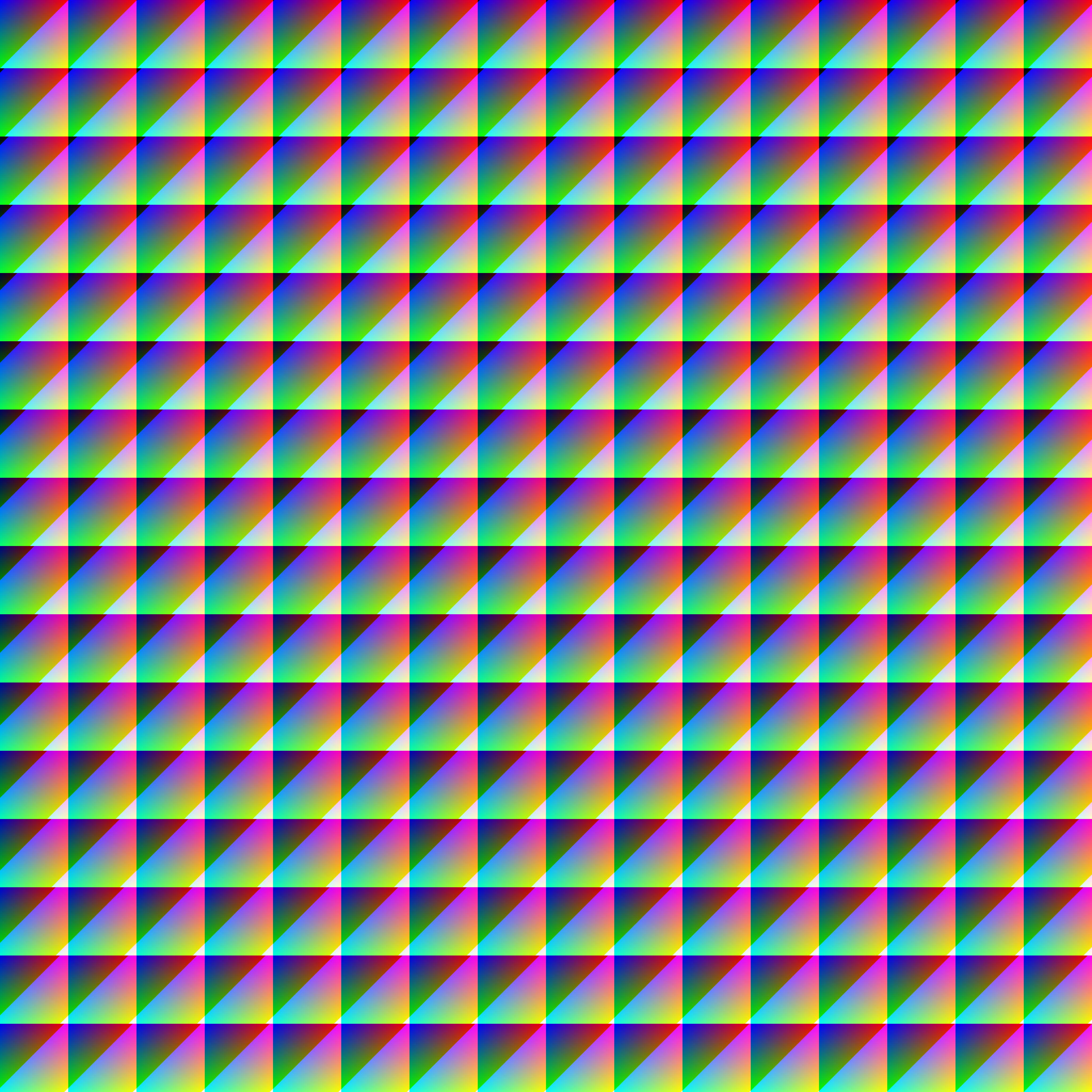# Value Layers

## Description

This shows all 766 value layers (sections where coordinate sums are equal) of the 256 edged cube. It is composed of 256 square segments like the "Thingy" image, but in this case 254 of the squares contain 3 layers (2 triangles and a hexagon) and the remaining 2 squares, in the upper left and lower right, contain 2 triangles each. In a typical cube these would all be regular polygons, but for the purposes of this square image, it is as if a distorted cube was sectioned, giving polygons that have 45, 90, and 135 degree angles.

## Stats

Date November 30, 2013 16,777,216 16,777,216 4,096 × 4,096 177,218